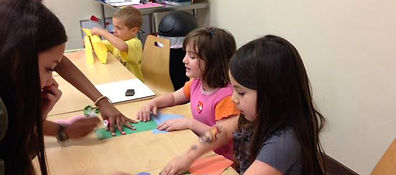top of page## Math Monkey Knowledge Center has classes for kids 3-14 years old

Students may start class at any time.  We believe in placing a child into a class by their ability, not by their age or grade.  The grade levels that are listed are recommendations only.  Click below to schedule a Free Assessment and Trial Class for your child.

Through our program your child will develop skills that take the fear out of math and allow for a new way to approach problems that complements math taught in school. Learning exciting new ways to look at math, both students looking for new challenges in math and students struggling in the subject benefit from the Math Monkey program.

As students progress through the program, techniques are introduced for problem solving. This progression continues to develop a strong number sense in the student, allows for critical thinking, and assists each student in attaining a soaring confidence in math.

They become masters at math and the results are seen in a renewed excitement for the subject, better grades and higher standardized test scores.

In just one session a week you will see your child’s abilities and understanding of math grow. Math Monkey is taught in a small class setting, allowing students to interact and learn from each other while still gaining personal attention from the teachers. For students that need a more personal approach, Math Monkey offers specific one-on-one tutoring from our trained assistants in addition to our weekly classes.

1. Critical Thinking & Problem Solving
2. Understanding OVER memorization
3. Learning should be a FUN and engaging process
4. Perfect compliment to school##### Pygmy Marmoset - Pre-School

Our youngest troop of pre-schoolers learn an introduction to basic math concepts such as number identification, counting, patterns, shapes and more ideas that will help support them in Pre-Kindergarten.

This is an activity-based math enrichment program filled with games and art projects that will stimulate and excite your child as they become develop a love for math.##### Green Monkey - Pre-K

Students continue to learn to read and write digits 0-9, develop an understanding of quantity (more/less) and master the basics of addition, subtraction, coins, clocks, shapes and story problems.

Students work on one-to-one correspondence, number recognition, Complements of 10, addition, subtraction, skip counting and developing a life-long love of math. Class sizes are kept to a minimum, never exceeding 10 children.##### Lemur - Kindergarten

Students will be introduced to beginning math concepts, especially using their mental math skills.  Some of the curriculum will include addition, subtraction, shapes, the number line, reading graphs and other tools to set them up for math success at an early age.

Students understand basic single digit addition and subtraction through 10, with little to no use of objects or fingers, comparison of objects, patterns, clock, coins and skip counting.

Recommended for Kindergarten – 1st Grade##### Spider Monkey - 1st Grade

Students focus on single and double digit addition and subtraction without fingers or objects, simple time and money skills, inequalities, introduction to fractions and measurement and many other tools to get them prepared for more logical mental math thought processes.

Students understand basic single digit addition and subtraction through 18 with little to no use of objects or fingers, adding and subtracting double digits with no carries or borrows, measurement, adding and subtracting using Complements of 10, missing addends, clock and money.Students will be introduced to traditional and mental math techniques for addition and subtraction, concepts of multiplication, advanced time and money, and word problems to increase their mental agility and create an even more positive attitude toward math.

Students understand Complements of 10 when adding and subtracting, Traditional vs. Mental addition with carries, Borrowing vs. Lending subtraction, time, money, measurement, graphs, line segments, perimeter, small word problems and patterns leading to multiplication.Students will continue to explore traditional and mental math techniques to increase their computational speed and accuracy.  They will be introduced to pre-algebra techniques using negative numbers, borrowing vs. lending subtraction, large addition problems using traditional vs. mental approaches, multiplication tables and a road map for learning how to solve more complicated word problems.

Students work on different techniques to add, subtract and multiply mentally, an intro to negative numbers, times tables, rounding, estimating, units of measurement, perimeter and area, Magic of 11 and in-depth word problem solving.Students will concentrate on double-digit and above multiplication, fractions, all mental addition and subtraction and other skills to help them explore higher-order thinking possibilities.  Students are challenged with logic puzzles and solve word problems with multiple concepts presented.

Students work on different advanced techniques to add, subtract and multiply mentally, subtraction using negative numbers, digit sums to check work, fractions, ratios, decimals, traditional long division, Nikhilam multiplication, percents, graphing, perimeter and area.By using their previous learning to work on higher level math problems, the students in Gorilla classes are ready to move on to experimenting with different mathematical concepts which will lead to critical thinking skills and advanced number sense.  The curriculum includes upper level fractions, percentages, ratios, triple-digit and above multiplication, division, pre-algebra, and many other advanced math concepts.Students in the Silverback troop concentrate their time on pre-algebra, geometry and critical thinking skills. This group continues to work on speed and accuracy with mental computations.  The curriculum includes word problems, order of operations, work with fractions and decimals, and exponents.##### Great Ape / Pre-Algebra

Great Ape classes prepare students for higher level math classes, as it integrates multiple math disciplines and allows the students to develop analysis and critical thinking skills.  These students work on algebra, geometry, data analysis, advanced order of operations as well as many other upper-level concepts that will prepare them for full high school Algebra.